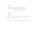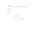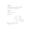# Exercise sheet 1 pdfLoading previews...first order differential equationseparable first order differential equationseparable first order differential equationhomogeneous first order differential equationseparable first order differential equation
10 files in this resource

## Exercise sheet 1 pdf

Exercises and solutions in PDFView Item